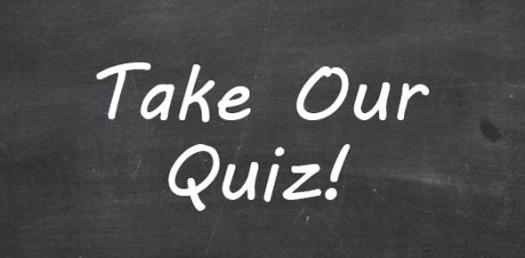Lesson 1 Quiz: Parts Of A Map Vocabulary

12 Questions | Total Attempts: 65SettingsEach of the following questions reviews key vocabulary terms for basic parts of a map. Read each question and select the correct answer. If you do not receive a 100%, re-study the vocabulary and re-take the quiz.

• 1.
This is 0 degrees longitude, it creates the Eastern and Western Hemisphere
• A.

Equator

• B.

Latitude

• C.

Prime Meridian

• D.

Map key

• 2.
Objects used to represent something else; usually a dot represents a city.
• A.

Key

• B.

Scale

• C.

Longitude

• D.

Symbols

• 3.
This is a tool used to measure distances on a map to compare to distances on the earth's surface.
• A.

Equator

• B.

Scale

• C.

Title

• D.

Labels

• 4.
This is a tool on a map showing cardinal (N,E,S,W) and intermediate (NE, NW, SE, SW) directions.
• A.

Compass rose

• B.

Legend

• C.

Scale

• D.

Labels

• 5.
This identifies the subject of the map.
• A.

Scale

• B.

Compass rose

• C.

Symbols

• D.

Title

• 6.
This explains the meaning of symbols on a map.
• A.

Compass rose

• B.

Legend/key

• C.

Scale

• D.

Title

• 7.
These are imaginary lines that run north and south across the earth.
• A.

Lines of longitude

• B.

Equator

• C.

Lines of latitude

• D.

Scale

• 8.
These are imaginary lines that circle the earth east to west.
• A.

Prime Meridian

• B.

Lines of longitude

• C.

Lines of latitude

• D.

Intermediate directions

• 9.
This is 0 degrees latitude, it divides the earth into the Northern and Southern hemisphere.
• A.

Lines of longitude

• B.

Symbols

• C.

Prime Meridian

• D.

Equator

• 10.
The directions of north, south, east and west are known as:
• A.

Symbols

• B.

Intermediate directions

• C.

Cardinal directions

• D.

Scale

• 11.
The directions of northeast, northwest, southeast, and southwest are known as:
• A.

Legend/key

• B.

Equator

• C.

Cardinal directions

• D.

Intermediate directions

• 12.
These identify objects on a map, for example names of cities, countries, bodies of water, etc.
• A.

Symbols

• B.

Labels

• C.

Scale

• D.

KeyBack to top